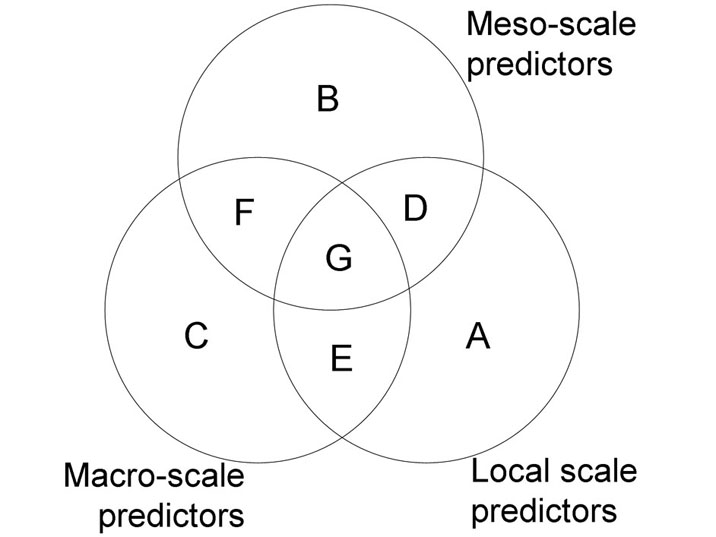### Jeffrey W. Matthews, Ariane L. Peralta, Diana N. Flanagan, Patrick M. Baldwin, Arun Soni, Angela D. Kent, and Anton G. Endress. 2009. Relative influence of landscape vs. local factors on plant community assembly in restored wetlands. Ecological Applications 19:2108–2123.

Appendix A. Procedure used to partition the variation in wetland vegetation composition among three sets of explanatory variables.

This appendix describes the procedure used to partition the variation in wetland vegetation composition among three sets of explanatory variables representing local, meso-, and macro-scale factors. The total explained variation was determined using a constrained ordination that included all nine explanatory variables (reduced from an initial set of 15 using the forward selection procedure in CANOCO 4.5). We then performed a series of 12 constrained ordinations (Table A1) to partition total variation (total inertia) in the species matrix into seven components (A, B, C, D, E, F, and G; Fig. A1) plus unexplained variation. The sum of the canonical eigenvalues from each constrained ordination corresponds to the amount of variation in the species data attributable to that set of explanatory variables after partialling out the effect of covariables. The proportion of the total variation explained by the explanatory variables in each ordination run was determined by dividing the sum of the canonical eigenvalues by the total inertia. The independent effect of local predictors (i.e., the amount of variation explained by local predictors alone, after partialling out the effects of meso- and macro-scale predictors) corresponds to component A (Fig. A1, Table A1), and the independent effects of meso- and macro-scale predictors correspond to components B and C, respectively. The amount of variation shared among sets of explanatory variables (joint effects; D, E, F, and G) was calculated algebraically based on the known partial effects. For example, component D, the variation shared between local and meso-scale predictors, independent of macro-scale predictors, was calculated by: (1) determining the independent effect of local plus meso-scale predictors (A + B + D) using a partial constrained ordination with macro-scale predictors specified as covariables, (2) determining the independent effect of local predictors alone (A) using a partial constrained ordination with meso- and macro-scale predictors as covariables, (3) determining the independent effect of meso-scale predictors (B) using a partial constrained ordination with local and macro-scale predictors as covariables, (4) subtracting the sums of canonical eigenvalues ([A + B + D] – A – B). The variation shared among the three sets of predictors (G) can be determined as:

 G = (A + D + E + G) – A – D – E, or G = (B + D + F + G) – B – D – F, or G = (C + E + F + G) – C – E – F.

TABLE A1. Variation partitioning using partial ordinations to determine the relative influence of macro-scale, meso-scale and local explanatory variables on species composition in restored wetlands.

 Main variables Covariables Components of variation Σ canonical eigenvalues Percent variation explained Total effect macro, meso, local A + B + C + D + E + F + G 2.96 45.2 Partial effects 1: local and macro + meso local macro, meso A 0.98 15.0 macro, meso B + C + D + E + F + G 1.98 30.2 macro, meso local B + C + F 1.69 15.8 local A + D + G + E 1.27 19.4 Partial effects 2: meso and local + macro meso local, macro B 0.50 7.6 local, macro A + C + D + E + F + G 2.46 37.6 local, macro meso A + C + E 2.18 33.2 meso B + D + F + G 0.78 12.0 Partial effects 3: macro and local + meso macro local, meso C 1.01 15.4 local, meso A + B + D + E + F + G 1.95 29.7 local, meso macro A + B + D 1.50 22.9 macro C + E + F + G 1.46 22.3 Joint effects meso and local D 0.02 0.4 local and macro E 0.19 2.8 macro and meso F 0.18 2.7 local, macro and meso G 0.08 1.3

Notes: Components of variation refer to letters in Fig. A1. Percent variation explained is the sum of the canonical eigenvalues divided by the total inertia (6.55).FIG. A1. Venn diagram illustrating percentages of variation in a species matrix that is attributable to three sets of explanatory variables: local, meso-scale, and macro-scale. The overlap of the circles represents variation shared between and among sets of explanatory variables. Letters correspond to percentages of variation explained by each component of variation.

[Back to A019-088]